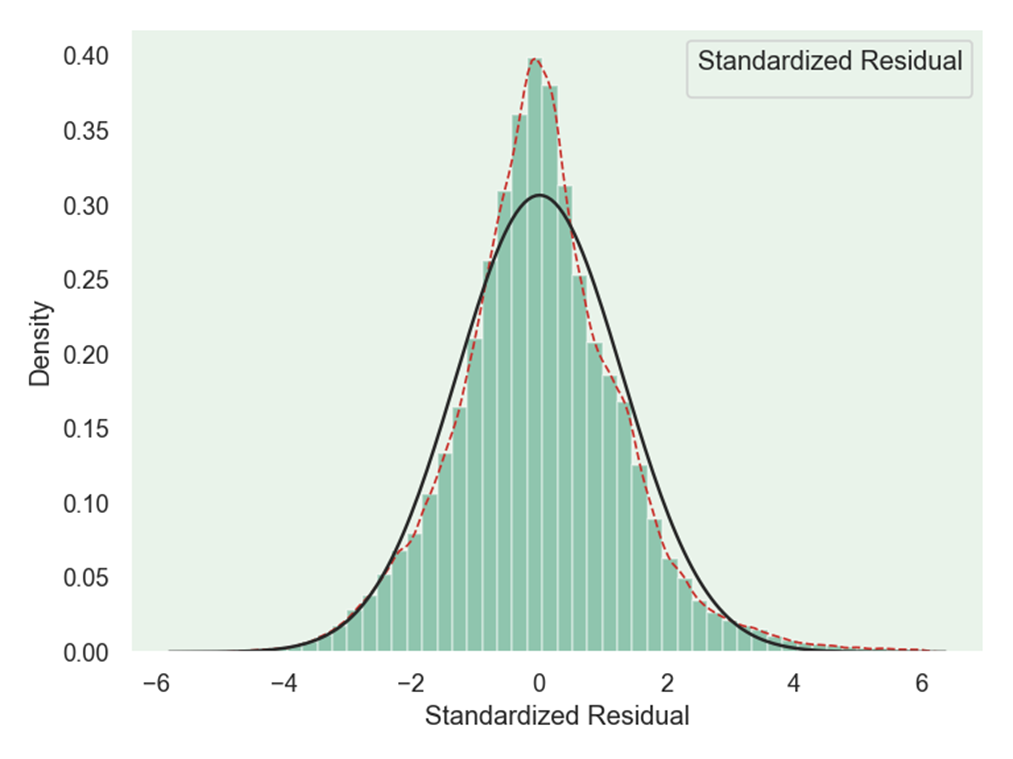• python画概率分布图
千次阅读
2019-10-29 17:49:08
import seaborn as sns

sns.kdeplot(df, shade = True)

更多相关内容
• # -*- coding: utf-8 -*- """ Created on Thu Oct 21 10:07:55 2021 @author: Machi """ import pandas as pd import seaborn as sns df = pd.read_csv('BSdata.csv') sns.distplot(df['身高'],kde = True, bins ...
# -*- coding: utf-8 -*-
"""
Created on Thu Oct 21 10:07:55 2021

@author: Machi
"""

import pandas as pd
import seaborn as sns
import matplotlib.pyplot as plt

plt.rcParams['font.sans-serif'] = ['SimSun']
sns.distplot(df['身高'],kde = True, bins = 20,rug = True)展开全文开发语言 后端
• Python绘制概率分布直方 文章目录Python绘制概率分布直方一、代码1、案例一2、案例二二、运行效果1、案例一2、案例二 一、代码 1、案例一 import random import numpy as np import matplotlib.pyplot as plt X...

## Python绘制概率分布直方图

### 一、代码

#### 1、案例一

import random
import numpy as np
import matplotlib.pyplot as plt

X = np.random.normal(loc=10, scale=2, size=(1, 1000))

Y = np.random.normal(loc=10, scale=1.0, size=(1, 1000))

P = np.ones(1000)
P[:600] = 0
np.random.shuffle(P)
# print(P)

Z = X + Y * P

plt.hist(Z, density=True, bins=150)
plt.show()



#### 2、案例二

import random
import numpy as np
import matplotlib.pyplot as plt

n = 1000
# p = 0.4
U = []

for i in range(1000):

X = np.random.normal(loc=10, scale=2, size=(1, n))

Y = np.random.normal(loc=10, scale=1.0, size=(1, n))

P = np.ones(n)
P[:600] = 0
np.random.shuffle(P)

Z = X + Y * P

a = 1 / pow(n * np.var(Z), 1/2)

b = sum(Z) - n * np.mean(Z)

u = a * b

U.append(u)

plt.hist(U, density=True, bins=60)
plt.show()



### 二、运行效果

#### 1、案例一#### 2、案例二最后，感谢大家的阅读与支持，谢谢大家了啦。

展开全文深度学习 机器学习
• 当我们想要弄清楚变量的统计特性时，往往想知道它是服从什么分布的，这时候就需要绘制概率分布直方python中我们可以使用seaborn库来进行绘制： Seaborn是一个基于matplotlib的Python数据可视化库。它为绘制有...

当我们想要弄清楚变量的统计特性时，往往想知道它是服从什么分布的，这时候就需要绘制概率分布直方图

在python中我们可以使用seaborn库来进行绘制：

Seaborn是一个基于matplotlib的Python数据可视化库。它为绘制有吸引力和信息丰富的统计图形提供了高级界面。

首先需要导入seaborn库：

import seaborn as sns


在seaborn中的distplot函数可以完成概率分布直方图和密度图的绘制

seaborn.distplot(a, bins=None, hist=True, kde=True, rug=False,
fit=None, hist_kws=None, kde_kws=None, rug_kws=None, fit_kws=None,
color=None, vertical=False, norm_hist=False, axlabel=None,
label=None, ax=None)


下面直接给出我绘制时用到的代码：

import seaborn as sns
import matplotlib as mpl
import matplotlib.pyplot as plt
from scipy.stats import norm
from scipy.stats import laplace

txt=[]
data=[]
path = r"E:\rtklib\rtklib-test1\stav.txt"

#mpl.rc("figure", figsize=(9, 5))
with open(path, "r") as f:

for i in range(len(txt)):
data.append(float(txt[i].strip().split(',')))

sns.set_palette("hls")
#sns.set_style("whitegrid")
plt.figure(dpi=120)
sns.set(style='dark')
sns.set_style("dark", {"axes.facecolor": "#e9f3ea"})
g = sns.distplot(data,
hist=True,
kde=True,  # 开启核密度曲线kernel density estimate (KDE)
kde_kws={'linestyle': '--', 'linewidth': '1', 'color': '#c72e29',
# 设置外框线属性
},
fit=norm,
color='#098154',
axlabel='Standardized Residual',  # 设置x轴标题

)

plt.show()


其中，distplot的参数中，kde表示是否绘制核密度曲线；fit是选择拟合的分布，来分析数据究竟是符合什么分布，seaborn中提供了很多分布，可以在这里找到，使用时如上我的代码中导入的那样就可以：连续分布类型
最后的效果图如下：绘制过程中没有加入太多修饰，如果想要好看一点，可以修改一些参数：
其中一个有设置背景 的风格，主要有以下几种：

sns.set_style("whitegrid")
sns.set_style("dark")
sns.set_style("darkgrid")
sns.set_style("white")
sns.set_style("ticks")

展开全文算法
• 目录   1、scipy库中各分布对应的方法   2、stats中各分布的常用方法及其功能   3、正态分布概率密度函数及其图象 ...    3）python绘制t分布和正态分布概率密度函数对比   6、F分布概率密度函数
• 今天小编就为大家分享一篇在python正态分布图像的实例，具有很好的参考价值，希望对大家有所帮助。一起跟随小编过来看看吧
• for i in sorted(list(set(df['yearmonth']))): df_sort = df[df['yearmonth'] == i].groupby('cust_id')['y','pred'].sum().sort_values('y',ascending = False) df_sort['yearmonth'] = i ...
• scale=1.5,size=I)#正态分布 x_logn = npr.lognormal(mean=0.5,sigma=1,size=I)#对数正态分布 x_chis = npr.chisquare(df=4,size=I)#卡方分布 x_beta = npr.beta(a=2,b=4,size=I)#贝塔分布 plt.figure(figsize =(12,...
• 主要介绍了Python绘制的二项分布概率图,涉及Python基于numpy、math的数值运算及matplotlib图形绘制相关操作技巧,需要的朋友可以参考下
• 主要介绍了python 计算概率密度、累计分布、逆函数的例子,具有很好的参考价值，希望对大家有所帮助。一起跟随小编过来看看吧
• 相应的概率分布有二项分布，泊松分布。连续型随机变量如果随机变量X的所有取值无法逐个列举出来，而是取数轴上某一区间内的任一点，则称X为连续型随机变量。相应的概率分布有正态分布，均匀分布，指数分布，伽马分布...
• 本文实例讲述了Python实现的概率分布运算操作。分享给大家供大家参考，具体如下： 1. 二项分布（离散） import numpy as np from scipy import stats import matplotlib.pyplot as plt ''' # 二项分布 (binomial ...
• 使用numpy和matplotlib.pyplot绘制正态分布、两点分布、二项分布 、几何分布、均匀分布 、指数分布曲线
• 四、用Python代码实现常见概率分布 二项分布（伯努利分布） from scipy import stats as st import numpy as np import matplotlib as mpl import matplotlib.pyplot as plt #防止乱码 mpl.rcParams['font.sans-...机器学习
• 概率分布表示样本数据的模样，长的好不好看如果有图像展示出来就非常完美了，使用Python绘制频率分布直方非常简洁，因为用的频次非常高，这里记录下来。还是Python大法好，代码简洁不拖沓~ 如果数据取值的范围跨度...
• 关于Python绘制正态分布图概率密度分布）以及遇到的问题（label无法显示） 目的：统计神经网络模型的参数分布情况 使用seaborn给我们提供的distplot函数来绘制，即调用sns.distplot()，并传入相关参数即可，这里...数据挖掘 深度学习
• 编辑：Peter转自：机器之心机器学习有其独特的数学基础，我们用微积分来处理变化无限小的函数，并计算...与此同时，从更细的角度来说，随机变量的概率分布也是我们必须理解的内容。在这篇文章中，项目作者介绍了所...机器学习 人工智能 大数据
• ## python画分布、密度等图形

万次阅读 多人点赞 2016-11-29 11:43:47
# jointplot还是非常实用的, 对于两个连续型变量的分布情况, 集中趋势能非常简单的给出. # 比如下面这个例子 x = stats.gamma(2).rvs(5000) y = stats.gamma(50).rvs(5000) with sns.axes_style("dark"): sns....
• matlab作概率密度函数很简单，但是本人学的python，因此想用python作图，从txt文本中读取数据，然后用python概率密度函数图像，
• 累积分布函数(Cumulative Distribution Function)，又叫分布函数，是概率密度函数的积分，能完整描述一个实随机变量X的概率分布。在电子器件制造中常常用于描述器件失效分布，可以明显的表示出在特定数值下的器件...
• 一.随机变量随机变量是指随机事件的数量表现，按照随机变量可能取得的...离散型随机变量的概率分布包括伯努利分布、二项分布、几何分布、泊松分布。连续型随机变量：在一定区间内变量取值有无限个，或数值无法一一列...
• 文章目录术语前言整数浮点数抽取字节洗牌排列贝塔分布二项分布卡方分布狄利克雷分布指数分布F分布伽玛分布几何分布耿贝尔分布超几何分布拉普拉斯分布（双指数分布）逻辑斯谛分布正态分布（高斯分布）对数正态分布...
• 今天小编就为大家分享一篇使用python绘制3维正态分布图的方法，具有很好的参考价值，希望对大家有所帮助。一起跟随小编过来看看吧
• 在下面是一个例子，说明如何用最初是一维的数据来处理这个问题(我使用随机值，如果是概率分布的话，应该对其进行规范化处理，但在这个例子中，这并不重要)：>>> import numpy as np>>> a = np....
• ## python累积分布图

千次阅读 2021-02-04 07:14:57
在与@EOL进行了决定性的讨论之后，我想使用随机高斯样本作为摘要发布我的解决方案(左上角)：import numpy as npimport matplotlib.pyplot as pltfrom math import ceil, floor, sqrtdef pdf(x, mu=0, sigma=1):""...
• 使用Python实现马尔科夫随机场、蒙特卡洛采样...因此本文介绍一下概率分布Python中定义的一种数据结构。一个概率分布的组成要素包含：随机变量、变量的维度、变量不同取值状态的对应概率值。在一个有向中(贝叶斯......